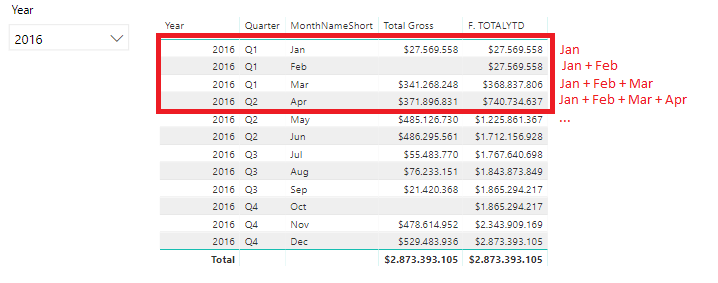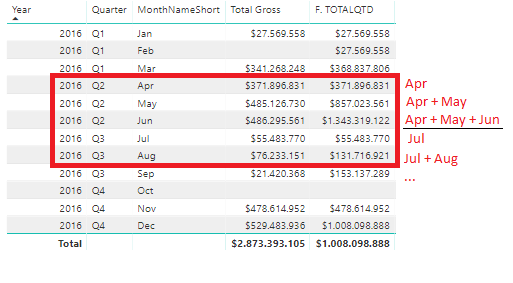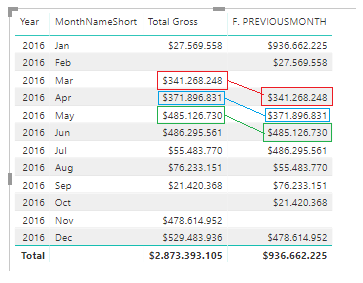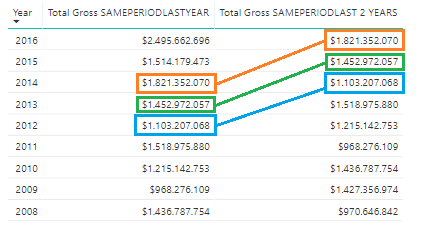One of the strengths of the DAX language is that it allows us to compare data for periods of time in a very simple and fast thanks to its intelligence functions of time. With the intelligence functions of time we can reduce a complicated query of T-SQL of 50 lines to a single line of code.

In this post we will describe the Time Intelligence Functions most used with their respective practical cases.

### 1. SAMEPERIODLASTYEAR

Probably it’s the most used intelligence time function. Through to this function, we can compare an amount between a period of time, with its amount in the same period of time but from the previous year. To use it we simply have to create a measurement, and in the filter part put the function SAMEPERIODLASTYEAR with the parameter of type date by which we want to compare, by default we will put the column of date type of our time dimension.

Once we present this measure to our client, the first thing he will indicate is that he wants the KPI of the improvement percentage with respect to the previous year. To make these measures in our case we will divide “Total Gross” of the selected period / “Total Gros” of the same period of the previous year.### 2. OTALYTD, TOTALQTD & TOTALMTD

When we talk about accumulated amounts we should always think about the following functions:

• #### TOTALYTD

This function evaluates the expression throughout the interval starting on the first day of the year and ending with the last date after applying the specified filters.• #### TOTALQTD

Evaluate the expression throughout the interval starting on the first day of the quarter and ending with the last date after applying the specified filters.• #### TOTALMTD

Like the two previous functions, it evaluates the expression throughout the interval starting at the beginning of the month and ending with the last date after applying the specified filters.

### 3. PREVIOUSDAY, PREVIOUSMONTH, PREVIOUSQUARTER & PREVIOUSYEAR

These functions, as the name indicates, return the information of the day, month, quarter or previous year.NEXTDAY, NEXTMONTH, NEXTQUARTER & NEXTYEAR are your equivalents for the next day, month, quarter or year.

### 4.DATEDIFF

Thanks to this function we can find out the interval of time between two dates.

The time intervals that this function can give us are the following:

• SECOND
• MINUTE
• HOUR
• DAY
• WEEK
• MONTH
• QUARTER
• YEAR

One of the practical cases where DATEDIFF is used is for the calculation of the average for a specific time interval, since, by default, the fact table is related to the time dimension by a column of type Date so that I would calculate the average per day. If we wanted to calculate the average per hour, the expression of the measure would be the following:

Whenever we want to add a time interval to a date, this is the function we should use. A practical case could be that a customer had a problem 2 years ago and would like to compare the current production with that of 2 years ago. To perform this measure we would use the SAMEPERIODLASTYEAR function but in the part where we add the parameter of the date, we would add the date but 2 years ago.Other functions that can also help us to perform DAX queries are the following:

TODAY: Returns the current date.
NOW: Returns the current date with hours, minutes and seconds.
FIRSTDATE: Returns the first date.
LASTDATE: Returns the last date.
FIRSTNONBLANK: Returns the first value that is not blank.
LASTNONBLANK: Returns the last value that is not blank.
DATESMTD, DATESQTD & DATESYTD: Returns a set of dates of the month, quarter or year to the current date.
ENDOFMONTH, ENDOFQUARTER & ENDOFYEAR: Returns the end of the month, quarter or year.
STARTOFMONTH, STARTOFQUARTER & STARTOFYEAR: Returns the beginning of the month, quarter or year.

### DimDate series:

Recommended Posts
•